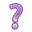## 16.2 Multi-level models

Here we specify separate models for the the fixed micro level effect of SES and the random macro level school effect.

\begin{equation*} \begin{aligned} MathScore_{ij} & = \beta_{0j} + \beta_{1}SES_{ij} + \epsilon_{ij}, & \qquad i = 1, \ldots, n_{j}\\ \beta_{0j} & = \gamma_{00} + U_{0j}, & \qquad j=1, \ldots, 23 \end{aligned} \end{equation*}

where

• $$\gamma_{00}$$ is the average intercept across all schools
• $$U_{0j}$$ is the difference between the intercept for school $$j$$ and the average intercept across all schools (random effect of school)

If we substitute the intercept $$\beta_{0j}$$ in the first model with the information from the second model,

$MathScore_{ij} = \overbrace{\gamma_{00} + \beta_{1}SES_{ij}}^{^{\mbox{fixed part}}} + \overbrace{U_{0j} + \epsilon_{ij}}^{\mbox{random part}}$

Instead of assuming that there is an overall average of math scores ($$\beta_0$$) from which each school deviates by a fixed amount, the above model assumes that the adjusted average school math scores are normally distributed and are centered at $$\gamma_{00}$$.

Because we assume that there is a distribution of intercepts, this model is also called a Random intercept model.

We’ll look at this type of model more closely in the next section using a different example, and using the concept of pooling information.Random Slopes

More complex models are possible. That’s a constant theme in all of statistical modeling. Just remember that

• the underlying complex effects being modeled must be strong enough that a simpler model that doesn’t account for these effects will be invalid
• you have to have enough data to estimate any additional parameters
• you have to be able to explain the model and results to an audience

We are not going to cover models that have random slopes, or interactions between fixed and random effects in this notebook.